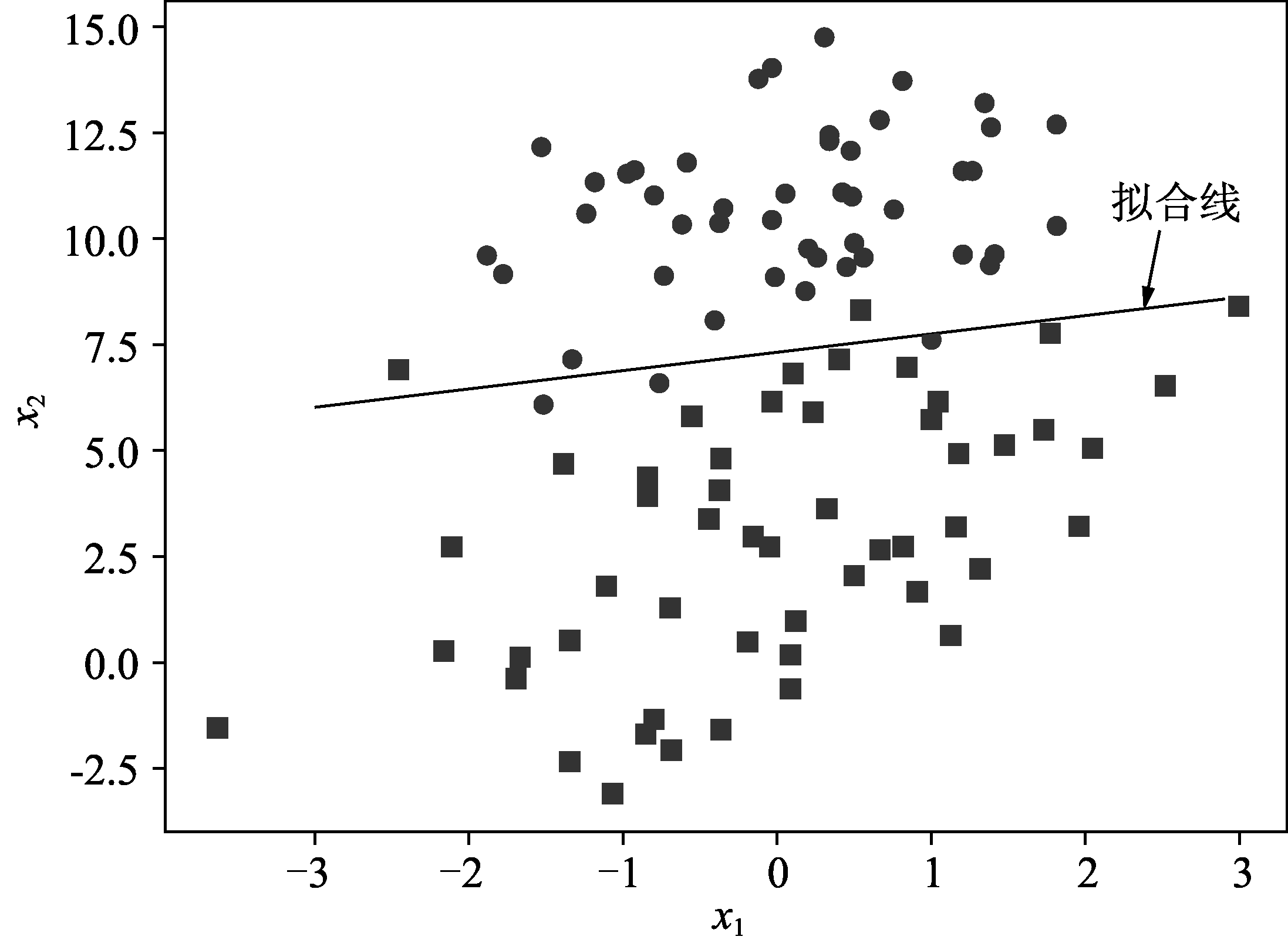# Python 数据挖掘与机器学习实战 (77)：回归分析介 3.7.4(数据预测)

from numpy import *import pandas as pdfrom pandas import DataFramefilename='/Users/apple27/Documents/data.txt'        #文件目录#df = DataFrame(pd.read_csv('/Users/apple27/Documents/logi.csv'))def loadDataSet():                #读取数据（这里只有两个特征）    df=pd.read_csv(filename)    print(df)    dataMat = []    labelMat = []    fr = open(filename)    for line in fr.readlines():        lineArr = line.strip().split()        dataMat.append([1.0, float(lineArr), float(lineArr)])#前面的 1 表示方程的常量。比如两个特征 X1 和 X2, 共需要 3 个参数#W1+W2*X1+W3*X2        labelMat.append(int(lineArr))    return dataMat,labelMat#调用函数loadDataSet()def sigmoid(inX):                  #定义 sigmoid 函数    return 1.0/(1+exp(-inX))def stocGradAscent1(dataMat, labelMat):#改进版随机梯度上升，在每次迭代中随机选择样本来更新权重#并且随迭代次数增加，权重变化越小    dataMatrix=mat(dataMat)    classLabels=labelMatm,n=shape(dataMatrix)    weights=ones((n,1))    maxCycles=500    for j in range(maxCycles):           #迭代        dataIndex=[i for i in range(m)]        for i in range(m):            #随机遍历每一行            alpha=4/(1+j+i)+0.0001           #随迭代次数增加, 权重变化越小            randIndex=int(random.uniform(0,len(dataIndex)))  #随机抽样            h=sigmoid(sum(dataMatrix[randIndex]*weights))            error=classLabels[randIndex]-h            weights=weights+alpha*error*dataMatrix[randIndex].transpose()            del(dataIndex[randIndex])       #去除已经抽取的样本return weights#画出最终分类的图def plotBestFit(weights):    import matplotlib.pyplot as plt    dataMat,labelMat=loadDataSet()    dataArr = array(dataMat)n = shape(dataArr)    xcord1 = []; ycord1 = []    xcord2 = []; ycord2 = []    for i in range(n):        if int(labelMat[i])== 1:            xcord1.append(dataArr[i,1])            ycord1.append(dataArr[i,2])        else:            xcord2.append(dataArr[i,1])            ycord2.append(dataArr[i,2])    fig = plt.figure()ax = fig.add_subplot(111)#定义颜色线条    ax.scatter(xcord1, ycord1, s=30, c='red', marker='s')ax.scatter(xcord2, ycord2, s=30, c='green')#坐标轴x = arange(-3.0, 3.0, 0.1)    y = (-weights-weights*x)/weightsax.plot(x, y)#绘图    plt.xlabel('X1')    plt.ylabel('X2')    plt.show()    plt.savefig('images/logExample.png', format='png'）def main():  #主函数    datamat,labelmat=loadDataSet()    weights=stocGradAscent1(datamat, labelmat).getA()    plotBestFit(weights)if __name__=='__main__':    main()